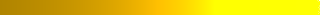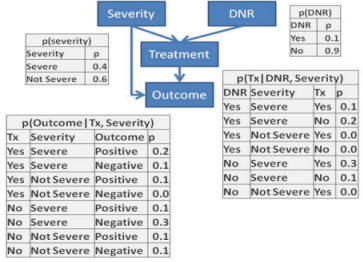# Lecture: Bayesian Networks

1. Introduction to probability networks Read► Slides►
2. Redundant models Read►
3. Comparison of Multivariate and Network Models Slides►
4. Fidelity between graph structure and formulas Slides►
5. Causal chain, common effect, and common cause in 3 variables Slides►
6. More on the relationship between independence, causality, and graph structure Video► Slides►
7. Propagation through a network using SQL Read► Video► Slides►
8. Learning Markov Blankets from independence tests Vang's Lecture► Reduce►
9. Learning network structure from Poisson regression Data► Read►

## Assignment

For this assignment you can use any statistical package, including R, SAS, and SPSS.  Your instructor is familiar with Netica and BayesiaLab.  R packages are also used often.  OpenBUGS and Gibbs Sampler, Stan, OpenMarkov, and Direct Graphical Model are open source software.  Netica is free for networks less than 15 nodes. A more complete list is available in Wikipedia under "Bayesian Networks."  OpenBUGS► Stan► Direct Graphical Models► OpenMarkov► Graphical Models Toolkit► PyMC► Genie Smile► SamIam► Bayes Server► AIspace► BayesiaLab► Hugin► AgenaRisk► dVelox► System Modeler► UnBBayes► Uninet► Tetrad► Dezide► Netica►

Work on this assignment can be done in group's of two students but you cannot work with a student that you have previously teamed up with.

1. Draw networks based on the following independence assumptions.  When directed networks are possible, give formulas for predicting the last variable in the networks from marginal and pair-wise conditional probabilities.  Keep in mind that absence of independence assumption implies dependence. Review►

 Nodes in Network Assumption X, Y, Z I(X,Y) X, Y, Z I(X,Y), Not I(X,Y|Z) X, Y, Z I(X,Y), I(X,Y|Z), Y measured last X, Y, Z, W I(X,Y), I(X,Y|Z), I({X,Y},W|Y), W measured last X, Y, Z, W I(X,Y), I(Z,W), and measured in the order given

Redo this assignment assuming that W, X, Y, Z occur in order, meaning W before X, X before Y, and Y before Z.  How have your networks changed?  Wang's Video►
2. Construct a Bayesian probability network model that would predict success with citalopram. A network model will include variables, and mediators of the effect of variables, on response to citalopram.   Include at least 5 variables in your model.  Remission or relapse should be considered an end node.  Variables that cannot be altered (e.g. year of birth or family history) should be considered root nodes.  All other  variables, e.g. diagnoses, could be either root or intermediary nodes. The structure of the network model could be based on expert's opinion, analysis of conditional independence, and your own analysis of association among the variables.  The probabilities among the variables should be estimated from the data.   Bushra's Response► Shruti's Video►Aras's Video►
3. Write an SQL code to calculate the probability of negative outcome in the situation where the patient is severely ill and has not signed a "Do Not Resuscitate" (DNR) order.  Note that probabilities for events that are mutually exclusive and exhaustive should add up to one. Data► Bushra's Response►Anto's Video► Slides► SQL►4. Redo problem 3 in Netica or other software and verify the accuracy of your answer.  To accomplish this project organize the 4 node network inside Netica and direct the links between the nodes as in the graph structure.  Then for every node, enter the table of probabilities as per tables given in Question 3.  For example, for the DNR node enter the two probabilities of 0.1 and 0.9 into the Table within the node for DNR.  Once the entire network (the graph and the related probabilities) has been entered into Netica, evaluate the risks for a patient who is severely ill and has not signed a "Do Not Resuscitate" order.     Netica► Shruti's Video►

## More

For additional information (not part of the required reading), please see the following links:

1. Meta analysis through Bayesian networks Read►
2. Introduction to Bayesian networks Read►
3. Learning Bayesian Networks Read►
4. Selection of Judea Pearl's articles PubMed►
5. Applications of Bayesian networks in healthcare PubMed►
6. Use of graphs in removing confounding Read►
7. Learning Bayesian networks from correlated data Read►
8. Bayesian networks in neuroscience Read►
9. Cost analysis using Bayesian networks Read►
10. Comparison of Bayesian network and logistic models Read►
11. Bayesian network classifiers Read►
12. Introduction to Markov process Tim's Lecture►
13. Explanation of predictions Aloudah's Lecture►
14. Outcome based prescribing for citalopram Slides►

This page is part of the course on Comparative Effectiveness by Farrokh Alemi, PhD Home► Email►